This comprehensive review of math problems for 4th graders is just the boost your child needs to succeed in math this year.Author
Amber WatkinsPublished
October 19, 2023This comprehensive review of math problems for 4th graders is just the boost your child needs to succeed in math this year.Author
Amber WatkinsPublished
Oct 19, 2023This comprehensive review of math problems for 4th graders is just the boost your child needs to succeed in math this year.Author
Amber WatkinsPublished
Oct 19, 2023Key takeaways

• In fourth-grade math, it is important to master multi-digit multiplication, fractions, and decimals.
• A variety of math problems for 4th graders can be solved using a number line.
• To supplement your child’s fourth-grade math skills, consider using our math app.

## 26 Math Problems for 4th GradersFourth-grade math has a strong focus on multiplication, division, and fractions. As we cover a variety of fourth-grade math problems together, pay attention to the methods we use, including breaking down fractions and using number lines to solve real-world word problems.

## Math Word Problems for 4th Graders: Multi-digit MultiplicationAnswer: Sam bought 144 bottles of water. There are 12 bottles in a dozen. 12 x 12 = 144.

Answer: Mr. Rodger bought 1,600 juice boxes. 100 x 16 = 1,600.

Answer: They bought 80 total binders. 6 x 5 = 30. 10 x 5 =50. 50 + 30 = 80.

Answer: Crystal has saved a total of 350 cents. Each dime is worth 10 cents. 35 x 10 =350.

Answer: Jason will have 720 new baseball cards after two years. There are 12 months in every year, so in two years there are 24 months (12 x 2 = 24). 24 x 30 =720.

## Word Problems for 4th Graders: Dividing to Find QuotientsAnswer: Jack should plant 14 seeds in each row. 98 / 7 = 14.

Answer: Kim should fill Polly’s dog bowl 8 times a day.  480 / 60 = 8

Answer: The sunflower has been growing for 12 weeks. There are 12 inches in a foot, so there are 24 inches in two feet (12 x 2 =24). 24 inches divided by 2 is equal to 12.

Answer: Cait will have to walk her dog 50 times to save \$500. 500 / 10 = 50

Answer: Jason will have 720 new baseball cards after two years. There are 12 months in every year, so in two years there are 24 months (12 x 2 = 24). 24 x 30 =720.

## 4th Grade Math Problems: FractionsAnswer: ⅛ + ⅛ + ⅛ + ⅛ + ⅛ + ⅛ + ⅛ + ⅛ = 6/8, so 8 people could have eaten ⅛ of a pie each or  ⅜ + ⅜ = 6/8, so 2 people could have eaten ⅜ of the pie each.

Answer: 1/9 + 1/9 + 1/9 + 1/9 + 1/9  or  3/9 + 6/9 = 5/9

Answer: Yes, they ran the same distance.

Answer: Yes they completed the same portion of their homework.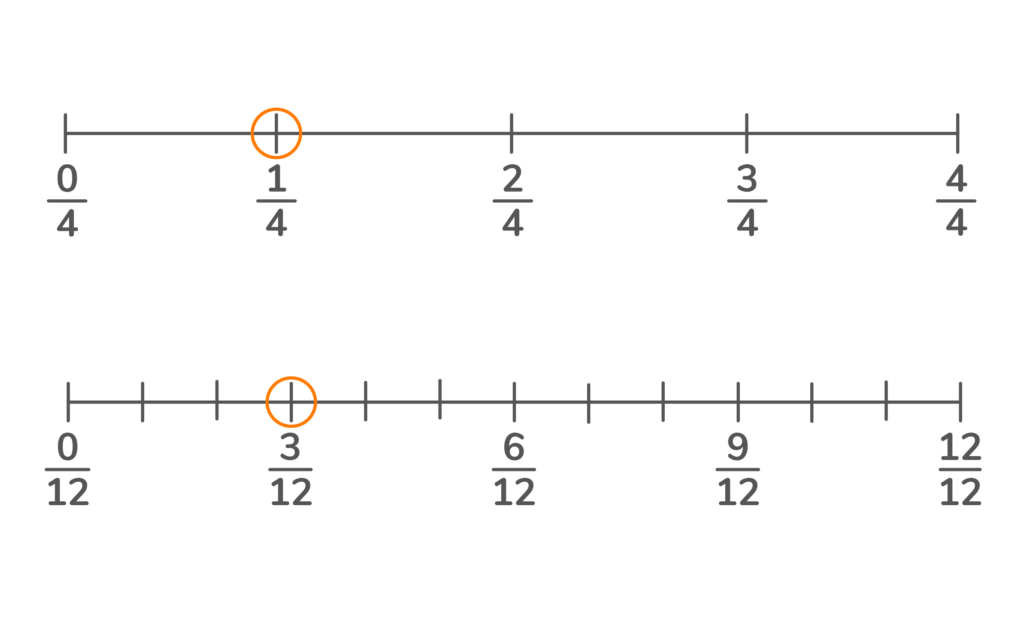Answer: The baker will need 6/8 cups of oil. ¾ x 2 = 6/8

Answer: She will need 1 ½ feet of space in her garden. 6 x 3/12 = 18/12 = 1 6/12 or 1 ½.

## Math Problems for 4th Graders: Rounding Whole Numbers using Number Lines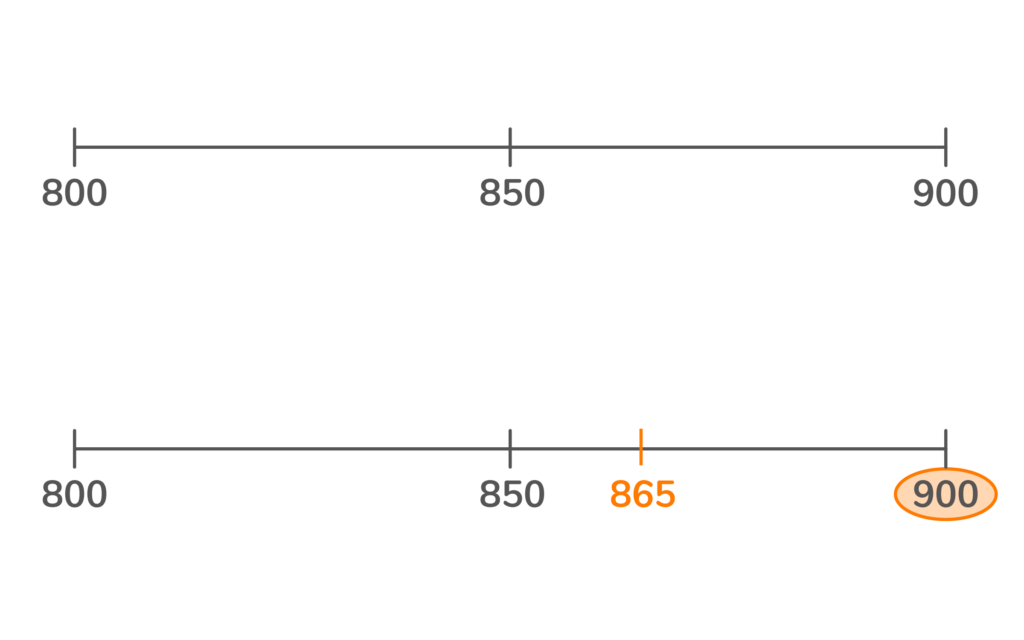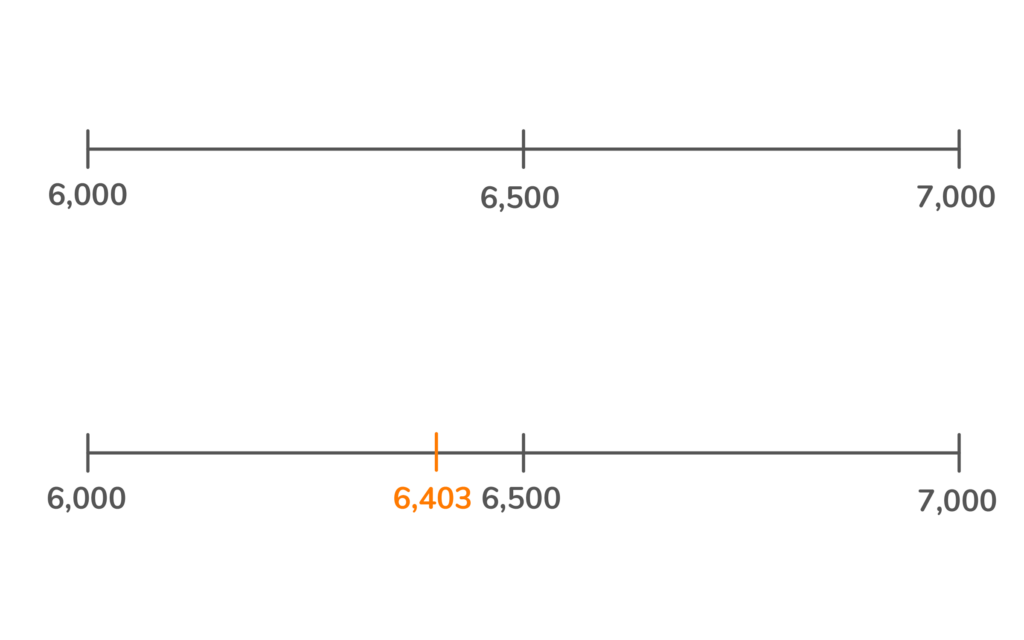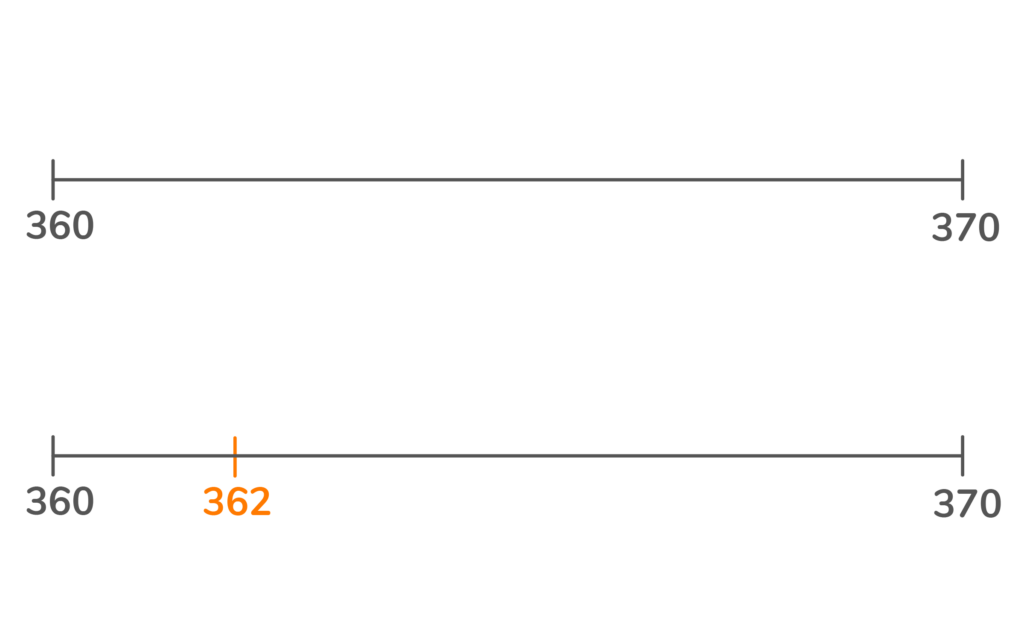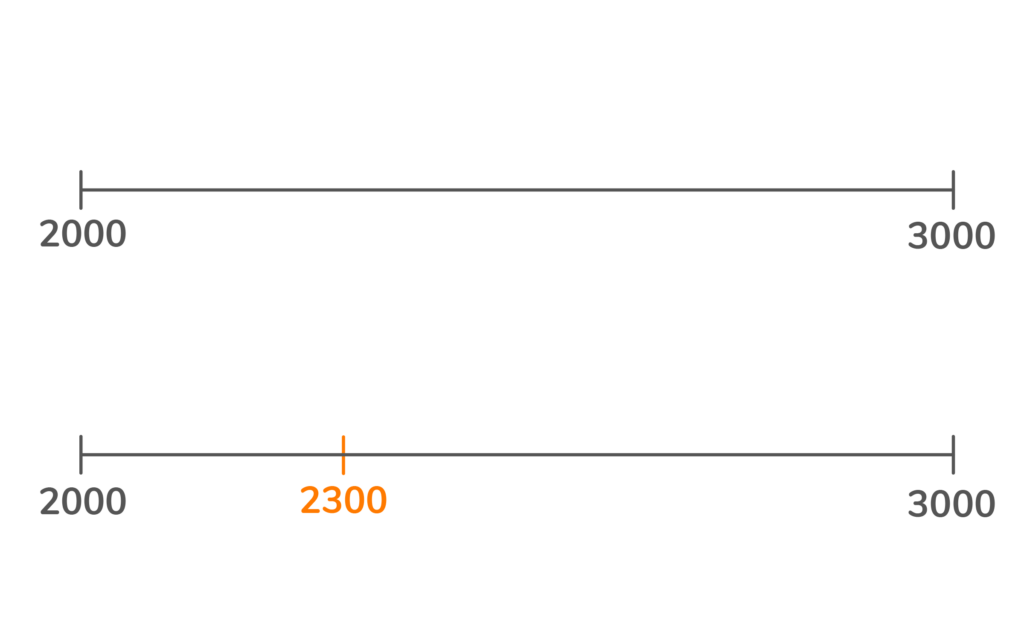## Math Problems for 4th Graders: Word Problems with Decimals and Money NotationAnswer: Jake’s grass will grow .7 millimetres in one week. .1 x 7 = .7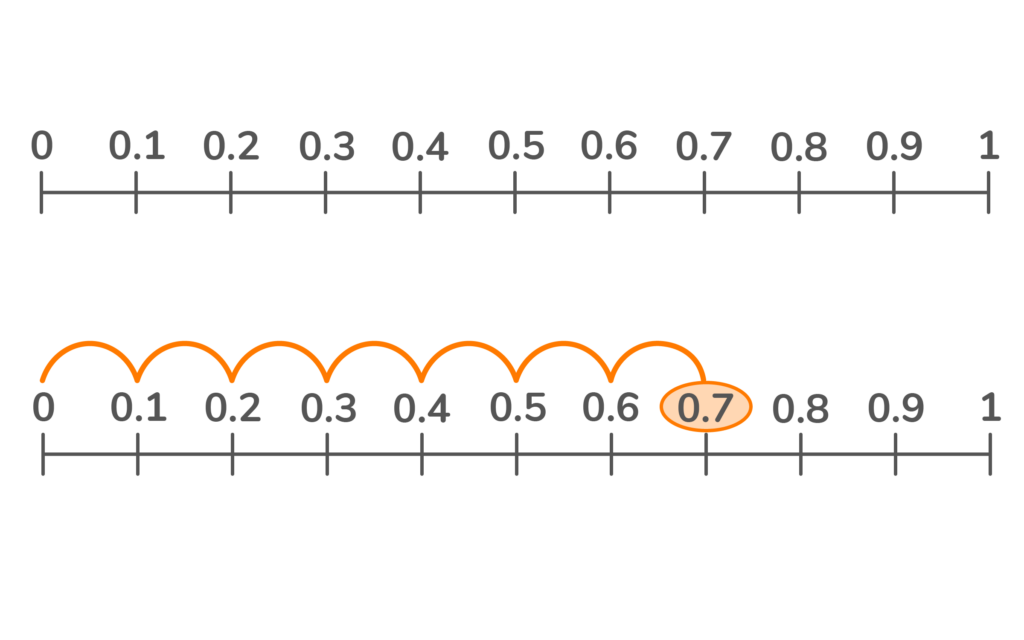Answer: Jason has a total of 60 cents (.60). A nickel is worth 5 cents or .05.

.05 x 12 is .60.

Answer: Reese spent a total of \$19.50 at the movie theater.

\$4.50 x 3 = \$13.50. \$3.00 x 2 = \$6.00. \$13.50 + \$6.00 = \$19.50

Answer: Steve has \$6.50 left over in his budget.
\$11.00 + \$12.50 + \$20.00 = \$43.50. \$50.00 – \$43.50 = \$6.50

Answer: Yes, he will have enough money for a \$200 deposit. \$52.12, \$69.40, \$42.80, and \$35.75 added together equal \$200.07.

Our math help app provides math help for fourth graders in a way that keeps children engaged, rewards their effort, and scaffolds their learning. Plus, the app gives parents access to a dashboard that shows their child’s progress on math topics they struggle with. Take learning into your own hands, and check out our math app today!

Lesson credits

Amber Watkins

Amber is an education specialist with a degree in Early Childhood Education. She has over 12 years of experience teaching and tutoring elementary through college level math. "Knowing that my work in math education makes such an impact leaves me with an indescribable feeling of pride and joy!"

Amber Watkins

Amber is an education specialist with a degree in Early Childhood Education. She has over 12 years of experience teaching and tutoring elementary through college level math. "Knowing that my work in math education makes such an impact leaves me with an indescribable feeling of pride and joy!"# Are you a parent, teacher or student?

Are you a parent or teacher?

## Hi there!

Book a chat with our team